Derivatives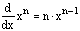The derivative of a power is the exponent times x to the power of the exponnent minus one.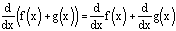The derivative of a sum is the sum of the derivatives of each function being added.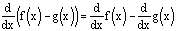The derivative of a difference is the difference of the derivatives of the functions being subtracted.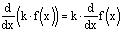The derivative of a constant times a function is the constant times the derivative of the function.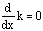The derivative of a constant is zero.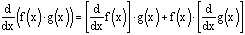The derivative of a product is the derivative of the first factor multiplied by the second factor plus the first factor multiplied by the derivative of the second factor.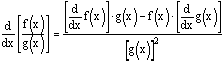The derivative of a quotient is the quotient of the derivative of the numerator multiplied by the denominator minus the numerator multiplied by the derivative of the denominator to the square of the denominator.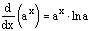The derivative of an exponential function is the product of the exponential function and the natural logarithm of the base.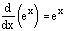Since the natural logarithm of e is 1, the derivative of e^(x) is e^(x).The derivative of a logarithm is 1 divided by the product of the natural logarithm of the base and x.The derivative of the natural logarithm of x is 1/x.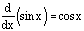The derivative of the sine of x is the cosine of x.The derivative of the cosine of x is the opposite of the sine of x.The derivative of the tangent of x is the square of the secant of x.The derivative of the secant of x is the product of the secant of x and the tangent of x.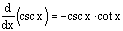The derivative of the cosecant of x is the opposite of the product of the cosecant of x and the cotangent of x.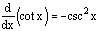The derivative of the cotangent of x is the opposite of the square of the cosecant of x.

Back to Formula